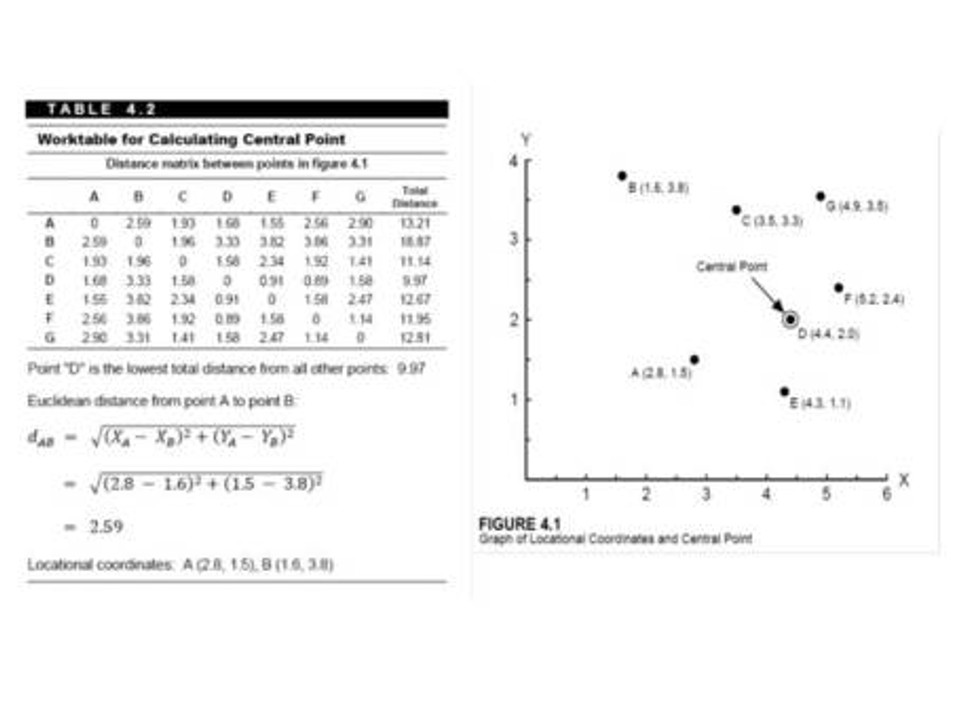Search

# Spatial is Not Special – Central Feature

This post is part of a series entitled Spatial is not Special, where I will illustrate how spatial constructs in SQL provide us with a rich toolset for geographers doing spatial analysis.  To illustrate these concepts, I will be showing examples from my book Statistical Problem Solving in Geography.  Even though PostGRES, SQLServer, MySQL, spatialite, and Oracle all support spatial SQL, these examples will be presented using Manifold GIS.  The example dataset is a Manifold 8.0 .map file and can be found here.

Our previous post showed how to extend the mean center of a geographic dataset to incorporate the weighted mean center using SQL.  Today’s post examines the SQL code necessary to generate the central feature for a geographic data set.  Recall from Statistical Problem Solving in Geography (third edition), the formula and computation of the Central Point.This is where the power of spatial SQL gets used.  As you can see, finding the central point requires us to compute a symmetrical matrix of the distance between each point and every other point. For our 7 point example, that’s 49 computations!  With spatial SQL, the distance function allows us to determine the distance between each of the points such as:

SELECT distance(a.id,b.id) AS dist FROM points a, points b

This SQL statement generates a table of distances between all the points – and we call the resulting column dist.  However, we need to determine the sum of all the distances for each point, and then select the lowest sum.  Because this SQL statement is a little complex, we will number each line and illustrate what is happening:

1. SELECT TOP 1 SUM(distance(a.id,b.id)) AS dist, a.id AS aid

2. FROM points AS a, points AS b

3. WHERE a.id <> b.id

4. GROUP BY a.id

5. ORDER BY dist

Line 1 selects the distances between each point and every other point.  However, because we have wrapped those distances in the SUM function, SQL will compute the sum of all the distances.  We have the TOP clause in this line, and will get back to its use in a moment.

Line 3 isn’t really necessary, but just ignores the computation of distances from each point with itself.

Line 4 is critical, because the SUM function is an aggregate clause, meaning it aggregates the values that are selected – in our case, it sums the values of the distances.  But, we don’t want the sum of ALL the distances, we want the sum of the distances aggregated by each of our 7  starting points.  Therefore, the GROUP BY clause is used to group the sum of the distances for each point ID.

Line 5 is also necessary because while we have the sum of the distances from each point to every other point, they aren’t in any particular order.  So, using the ORDER BY clause, we can essentially sort the sum of the distances from low to high.

Remember, at this point we have the sum of all the distances from each point to every other point sorted by the distance.  This means that the first record in the result is the point with the lowest sum of the distances to all other points, and the last record is the point with the highest sum of the distances.

We can now revisit Line 1, and the TOP clause.  If the first record returned (due to our ORDER BY clause in Line 5) is the lowest summed distance, then selecting the TOP 1 from that list obviously returns the feature with the lowest sum of all distances to all the other point.